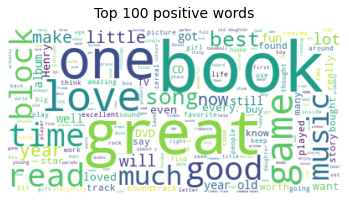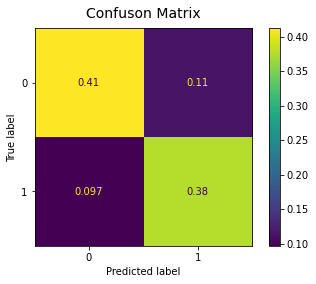Back to Templates

# Sentiment Analysis and Prediction

Sentiment analysis is the process of understanding the opinion of an author about a subject. Examples include analyzing movie ratings, amazon product reviews or the analysis of Twitter tweet sentiment.

• Explore our data
• Transform sentiment carrying columns
• Predict sentiment with a supervised machine learning model
```.css-y3yaiy{border:0;border-radius:4px;color:rgb(5, 25, 45);font-family:monospace;font-family:JetBrainsMonoNL;font-size:14px;font-variant-ligatures:none;letter-spacing:0;line-height:1.5;margin:0;padding:0;}```%%capture
!pip install wordcloud``````
``````# Imports
import matplotlib.pyplot as plt
import pandas as pd
import numpy as np
import nltk
from sklearn.metrics import accuracy_score, confusion_matrix, plot_confusion_matrix
from sklearn.linear_model import LogisticRegression
from sklearn.model_selection import train_test_split
from sklearn.feature_extraction.text import TfidfVectorizer, CountVectorizer, ENGLISH_STOP_WORDS
from wordcloud import WordCloud
from functools import reduce
from nltk import word_tokenize
``````[nltk_data] Downloading package punkt to /home/repl/nltk_data...
[nltk_data]   Unzipping tokenizers/punkt.zip.

True``````

Upload data that has textual value and an indication of the sentiment (0 = negative, 1 = positive)

``````# Upload your data as CSV and load as a data frame
scorereview
01Stuning even for the non-gamer: This sound tr...
11The best soundtrack ever to anything.: I'm re...
21Amazing!: This soundtrack is my favorite musi...
31Excellent Soundtrack: I truly like this sound...
41Remember, Pull Your Jaw Off The Floor After H...

## 2. Word cloud and feature creation

Visualize words that carry meaning with a word cloud

``````positive_df = df[df['score'] == 1]['review'][:100]             # 1 = positive, 0 = negative
positive_df = reduce(lambda a, b: a+b, positive_df)

# Create and generate a word cloud image
cloud_positives = WordCloud(background_color='white')                     .generate(positive_df)

# Display the generated wordcloud image
plt.imshow(cloud_positives, interpolation='bilinear')
plt.title('Top 100 positive words', y = 1.02, size = 14)       # Choose title, position and size
plt.axis("off")                                                # Turn off axis labels

# Don't forget to show the final image
plt.show()````````````# Tokenize each item in the review column
word_tokens = [word_tokenize(review) for review in df['review']]

# Create a new feature for the lengh of each review
df['n_words'] = [len(word_tokens[i]) for i in range(len(word_tokens))]

scorereviewn_words
01Stuning even for the non-gamer: This sound tr...87
11The best soundtrack ever to anything.: I'm re...109
21Amazing!: This soundtrack is my favorite musi...165
31Excellent Soundtrack: I truly like this sound...145
41Remember, Pull Your Jaw Off The Floor After H...109

## 3. Building a vectorizer

Use the Tfidf Vectorizer to transform the data into numerical values that can be used to make predictions.

capture only words using the specified pattern.

``````# Build the vectorizer
vect = TfidfVectorizer(stop_words=ENGLISH_STOP_WORDS,             # Default list of English stop words
ngram_range=(1, 2),                        # Consider Uni- and Bi-grams
max_features=200,                          # Max number of features
token_pattern=r'[^dW][^dW]+')      # Capture only words using this pattern

vect.fit(df.review)

# Create sparse matrix from the vectorizer
X = vect.transform(df.review)

# Create a DataFrame
df_transformed = pd.DataFrame(data=X.toarray(),
columns=vect.get_feature_names())
00.00.00.00.00.00.0000000.00.00.2740410.0...0.0000000.00.00.00.0000000.00.00.00.00.000000
10.00.00.00.00.00.0000000.00.00.0000000.0...0.0000000.00.00.00.2194080.00.00.00.00.208885
20.00.00.00.00.00.3827730.00.00.0000000.0...0.1429350.00.00.00.1600890.00.00.00.00.152410
30.00.00.00.00.00.0000000.00.00.0000000.0...0.0000000.00.00.00.0000000.00.00.00.00.000000
40.00.00.00.00.00.0000000.00.00.0000000.0...0.0000000.00.00.00.0000000.00.00.00.00.000000
``5 rows x 200 columns``

## 4. Building a classifier

Use a logistic regression to predict the sentiment of unseen data. Visualize the errors your classifier makes with a confusion matrix.

``````dropped = df.drop(['review', 'n_words'],axis=1)
transformed = pd.concat([dropped, df_transformed], axis=1)
scoreableactionactuallyagoalbumamazingamazonauthoraway...workworksworldworstworthwritingwrittenwrongyearyears
010.00.00.00.00.00.0000000.00.00.274041...0.0000000.00.00.00.0000000.00.00.00.00.000000
110.00.00.00.00.00.0000000.00.00.000000...0.0000000.00.00.00.2194080.00.00.00.00.208885
210.00.00.00.00.00.3827730.00.00.000000...0.1429350.00.00.00.1600890.00.00.00.00.152410
310.00.00.00.00.00.0000000.00.00.000000...0.0000000.00.00.00.0000000.00.00.00.00.000000
410.00.00.00.00.00.0000000.00.00.000000...0.0000000.00.00.00.0000000.00.00.00.00.000000
``5 rows x 201 columns``
``````# Define X and y
y = transformed['score']
X = transformed.drop('score', axis=1)

# Train/test split
X_train, X_test, y_train, y_test = train_test_split(X, y,
test_size=0.2,     # Set size of test_set
random_state=456)  # Random seed for reproducibility

# Train a logistic regression
log_reg = LogisticRegression().fit(X_train, y_train)

# Predict the labels
y_predicted = log_reg.predict(X_test)

# Print accuracy score and confusion matrix on test set
print('Accuracy on the test set: ', accuracy_score(y_test, y_predicted))
print(confusion_matrix(y_test, y_predicted)/len(y_test))``````
``````Accuracy on the test set:  0.789
[[0.412 0.114]
[0.097 0.377]]``````
``````plot_confusion_matrix(log_reg, X_test, y_test, normalize='all')
plt.title('Confuson Matrix', y=1.02, size=14)
plt.show() ``````### Sentiment Analysis and Prediction

Understand and predict the opinion of an author about a subject.

Use Template